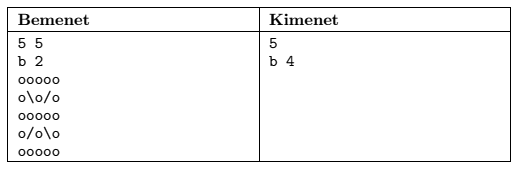Mathematical and Physical Journal
for High Schools
Issued by the MATFUND Foundation
 Already signed up? New to KöMaL?

#Problem I. 367. (February 2015)

I. 367. Some mirrors are placed diagonally in certain squares of a uniform square grid of size $\displaystyle M\times N$ ($\displaystyle 5\le M, N\le 100$). Both sides of the mirrors reflect the light perfectly, and a mirror can freely rotate about the center of the square. The light in this model travels one unit of distance (=the side length of a square) in one unit of time. A light impulse enters the grid perpendicular to one of its sides, and initially passes through the midpoint of the side of a square. In each instant when the light impulse hits the side of a square of the grid, the mirrors are rotated by 90 degrees.

The first line of the input file (Bemenet'' in the example) contains the number of rows and columns. The second line contains the incoming direction (b, j, f, l, meaning from left/right/top/bottom'') and position of the light impulse (the square positions are counted from top to bottom and from left to right). Then each of the following $\displaystyle M$ lines contains exactly $\displaystyle N$ characters: the space character (o in the example) denotes a square without a mirror, while the \ and / characters denote the initial state of the mirrors.The first line of the output file (Kimenet'' in the example) should contain the time needed for the impulse to leave the grid. The second line of the file should contain the exit position (using the same format as in the second input line). If the light is trapped within the grid, the first output line should contain a -1 value, and the second line should remain empty.

The first and second command line arguments of your program are the name of the input and output files, respectively.

The source code (i367.pas, i367.cpp, ...) of your program with a short documentation (i367.pdf) of your program and solution, also describing which developer environment to use for compiling the source, should be submitted in a compressed file i367.zip.

(10 pont)

Deadline expired on March 10, 2015.

### Statistics:

 11 students sent a solution. 8 points: 1 student. 6 points: 1 student. 5 points: 1 student. 4 points: 4 students. 3 points: 3 students. 2 points: 1 student.

Problems in Information Technology of KöMaL, February 2015